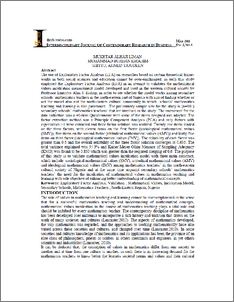# Exploratory factor analysis: aalidation of Mathematical Values Inculcation Model among secondary schools' mathematics teachers in North-Eastern region, Nigeria

Liman, Mukhtar Alhaji and Ibrahim, Mohd Burhan and Shittu, Ahmed Tajudeen (2011) Exploratory factor analysis: aalidation of Mathematical Values Inculcation Model among secondary schools' mathematics teachers in North-Eastern region, Nigeria. Interdisciplinary Journal of Contemporary Research in Business, 3 (1). pp. 64-72. ISSN 2073-7122Preview
PDF (IJCRB (May 2011)) - Published Version
Official URL: http://ijcrb.webs.com

## Abstract

The use of Exploratory Factor Analysis (EEA) on researches based on certain theoretical frame-works in both social sciences and education cannot be over-emphasized, as such this study employed the Exploratory Factor Analysis (EFA) in an attempt to validates the mathematical values inculcation measurement model developed and used in the western cultural society by Professor Emeritus Alan J. Bishop, in order to see whether the model works among secondary schools’ mathematics teachers in the north-eastern part of Nigeria with aim of finding whether or not the model also suit the north-eastern cultural community to which schools' mathematics teaching and learning is also paramount. The preliminary sample size for the study is (n=101) secondary schools’ mathematics teachers that are involved in the study. The instrument for the data collection was a 40-item Questionnaire with some of the items designed and adopted. The factor extraction method was a Principle Component Analysis (PCA) and only factors with eigenvalues >1 were extracted and three factor solution was realized. Twenty one items loaded on the three factors, with eleven items on the first factor (sociological mathematical values (SMV)) - five items on the second factor (attitudinal mathematical values (AMV)) and lastly five items on third factor (ideological mathematical values (IMV)). The reliability of each factor was greater than 0.6 and the overall reliability of the three factor solution converges at 0.636. The total variance explained was 54.3% and Kaiser-Meyer-Olkin Measure of Sampling Adequacy (KMO) was found to be 0.850 which was greater than the required merging of 0.6. The purpose of this study is to validate mathematical values inculcation model with three main construct, which include: sociological mathematical values (SMV). attitudinal mathematical values (AMV) and ideological mathematical values (IMV) among mathematics teachers'" in the north-eastern cultural society of Nigeria and at the same time acquaint secondary schools' mathematics teachers' the need for the inculcation of mathematical values in mathematics teaching and learning with sole objective of enhancing better understanding of mathematical concepts.

Item Type: Article (Journal) 6559/28811 Exploratory Factor Analysis; validation; mathematical values; Inculcation Models; secondary schools; mathematical teachers; North-Eastern region, Nigeria L Education > L Education (General) Kulliyyah of Education Dr Mohd Burhan Ibrahim 04 Apr 2013 08:45 04 Apr 2013 08:45 http://irep.iium.edu.my/id/eprint/28811View Item